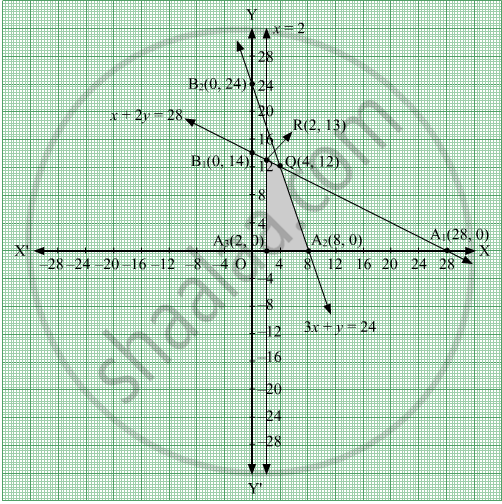Share

# Solve the Following L.P.P Graphically: Maximise Z = 20x + 10y Subject to the Following Constraints X + 2y ≤ 28, - CBSE (Science) Class 12 - Mathematics

ConceptGraphical Method of Solving Linear Programming Problems

#### Question

Solve the following L.P.P graphically: Maximise Z = 20x + 10y

Subject to the following constraints x + 2y ≤ 28,

3x + y ≤ 24,

x ≥ 2,

x, y ≥ 0

#### Solution

The given constraints are x + 2y ≤ 28, 3x + y ≤ 24, x ≥ 2 and x, y ≥ 0.

Converting the inequations into equations, we obtain the following equations:

x + 2y = 28, 3x + y = 24, x = 2, x = 0 and y = 0

These equations represents straight lines in XOY plane.

The line x + 2y = 28 meets meets the coordinate axes at A1(28, 0) and B1(0, 14). Join these points to obtain the line x + 2y = 28.

The line 3x + y = 24 meets meets the coordinate axes at A2(8, 0) and B2(0, 24). Join these points to obtain the line 3x + y = 24.

The line x = 2, is parallel to y-axis, passes through the point A3(2, 0).

Also, x = 0 is the y-axis and y = 0 is the x-axis.

The feasible region of the LPP is shaded below.The point of intersection of lines x + 2y = 28 and 3x + y = 24 is Q(4, 12).

The point of intersection of lines x = 2 and x + 2y = 28 is R(2, 13).

The coordinates of the corner points of the feasible region are A3(2, 0), A2(8, 0), Q(4, 12) and R(2, 13).

The values of the objective function at these points are given in the following table:

 Point Value of the objective function Z = 20x + 10y A3(2, 0) Z = 20 × 2 + 10 × 0 = 40 A2(8, 0) Z = 20 × 8 + 10 × 0 = 160 Q(4, 12) Z = 20 × 4 + 10 × 12 = 200 Maximum R(2, 13 Z = 20 × 2 + 10 × 13 = 170

Clearly, Z is maximum at Q(4, 12) and the maximum value of Z is 200.

Is there an error in this question or solution?

#### Video TutorialsVIEW ALL 

Solution Solve the Following L.P.P Graphically: Maximise Z = 20x + 10y Subject to the Following Constraints X + 2y ≤ 28, Concept: Graphical Method of Solving Linear Programming Problems.
S